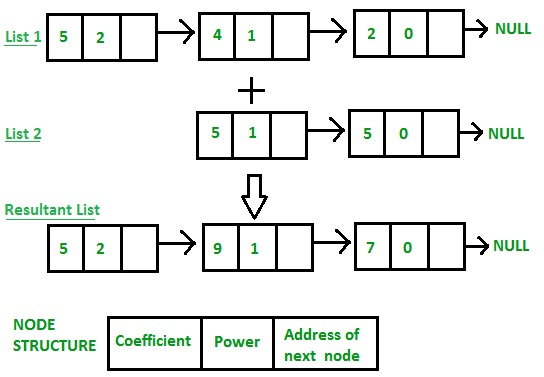Related Articles
• Difficulty Level : Medium
• Last Updated : 23 Apr, 2021

Given two polynomial numbers represented by a linked list. Write a function that add these lists means add the coefficients who have same variable powers.
Example:

```Input:
1st number = 5x2 + 4x1 + 2x0
2nd number = -5x1 - 5x0
Output:
5x2-1x1-3x0
Input:
1st number = 5x3 + 4x2 + 2x0
2nd number = 5x^1 - 5x^0
Output:
5x3 + 4x2 + 5x1 - 3x0```## CPP

 `// C++ program for addition of two polynomials``// using Linked Lists``#include ``using` `namespace` `std;` `// Node structure containing power and coefficient of``// variable``struct` `Node {``    ``int` `coeff;``    ``int` `pow``;``    ``struct` `Node* next;``};` `// Function to create new node``void` `create_node(``int` `x, ``int` `y, ``struct` `Node** temp)``{``    ``struct` `Node *r, *z;``    ``z = *temp;``    ``if` `(z == NULL) {``        ``r = (``struct` `Node*)``malloc``(``sizeof``(``struct` `Node));``        ``r->coeff = x;``        ``r->``pow` `= y;``        ``*temp = r;``        ``r->next = (``struct` `Node*)``malloc``(``sizeof``(``struct` `Node));``        ``r = r->next;``        ``r->next = NULL;``    ``}``    ``else` `{``        ``r->coeff = x;``        ``r->``pow` `= y;``        ``r->next = (``struct` `Node*)``malloc``(``sizeof``(``struct` `Node));``        ``r = r->next;``        ``r->next = NULL;``    ``}``}` `// Function Adding two polynomial numbers``void` `polyadd(``struct` `Node* poly1, ``struct` `Node* poly2,``             ``struct` `Node* poly)``{``    ``while` `(poly1->next && poly2->next) {``        ``// If power of 1st polynomial is greater then 2nd,``        ``// then store 1st as it is and move its pointer``        ``if` `(poly1->``pow` `> poly2->``pow``) {``            ``poly->``pow` `= poly1->``pow``;``            ``poly->coeff = poly1->coeff;``            ``poly1 = poly1->next;``        ``}` `        ``// If power of 2nd polynomial is greater then 1st,``        ``// then store 2nd as it is and move its pointer``        ``else` `if` `(poly1->``pow` `< poly2->``pow``) {``            ``poly->``pow` `= poly2->``pow``;``            ``poly->coeff = poly2->coeff;``            ``poly2 = poly2->next;``        ``}` `        ``// If power of both polynomial numbers is same then``        ``// add their coefficients``        ``else` `{``            ``poly->``pow` `= poly1->``pow``;``            ``poly->coeff = poly1->coeff + poly2->coeff;``            ``poly1 = poly1->next;``            ``poly2 = poly2->next;``        ``}` `        ``// Dynamically create new node``        ``poly->next``            ``= (``struct` `Node*)``malloc``(``sizeof``(``struct` `Node));``        ``poly = poly->next;``        ``poly->next = NULL;``    ``}``    ``while` `(poly1->next || poly2->next) {``        ``if` `(poly1->next) {``            ``poly->``pow` `= poly1->``pow``;``            ``poly->coeff = poly1->coeff;``            ``poly1 = poly1->next;``        ``}``        ``if` `(poly2->next) {``            ``poly->``pow` `= poly2->``pow``;``            ``poly->coeff = poly2->coeff;``            ``poly2 = poly2->next;``        ``}``        ``poly->next``            ``= (``struct` `Node*)``malloc``(``sizeof``(``struct` `Node));``        ``poly = poly->next;``        ``poly->next = NULL;``    ``}``}` `// Display Linked list``void` `show(``struct` `Node* node)``{``    ``while` `(node->next != NULL) {``        ``printf``(``"%dx^%d"``, node->coeff, node->``pow``);``        ``node = node->next;``        ``if` `(node->coeff >= 0) {``            ``if` `(node->next != NULL)``                ``printf``(``"+"``);``        ``}``    ``}``}` `// Driver code``int` `main()``{``    ``struct` `Node *poly1 = NULL, *poly2 = NULL, *poly = NULL;` `    ``// Create first list of 5x^2 + 4x^1 + 2x^0``    ``create_node(5, 2, &poly1);``    ``create_node(4, 1, &poly1);``    ``create_node(2, 0, &poly1);` `    ``// Create second list of -5x^1 - 5x^0``    ``create_node(-5, 1, &poly2);``    ``create_node(-5, 0, &poly2);` `    ``printf``(``"1st Number: "``);``    ``show(poly1);` `    ``printf``(``"\n2nd Number: "``);``    ``show(poly2);` `    ``poly = (``struct` `Node*)``malloc``(``sizeof``(``struct` `Node));` `    ``// Function add two polynomial numbers``    ``polyadd(poly1, poly2, poly);` `    ``// Display resultant List``    ``printf``(``"\nAdded polynomial: "``);``    ``show(poly);` `    ``return` `0;``}`

## Java

 `import` `java.io.*;``import` `java.util.Scanner;` `class` `Polynomial {``    ``public` `static` `Node addPolynomial(Node p1, Node p2)``    ``{` `        ``Node a = p1, b = p2, newHead = ``new` `Node(``0``, ``0``),``             ``c = newHead;` `        ``while` `(a != ``null` `|| b != ``null``) {` `            ``if` `(a == ``null``) {``                ``c.next = b;``                ``break``;``            ``}``            ``else` `if` `(b == ``null``) {``                ``c.next = a;``                ``break``;``            ``}` `            ``else` `if` `(a.pow == b.pow) {``                ``c.next = ``new` `Node(a.coeff + b.coeff, a.pow);` `                ``a = a.next;``                ``b = b.next;``            ``}` `            ``else` `if` `(a.pow > b.pow) {``                ``c.next = ``new` `Node(a.coeff, a.pow);` `                ``a = a.next;``            ``}` `            ``else` `if` `(a.pow < b.pow) {``                ``c.next = ``new` `Node(b.coeff, b.pow);` `                ``b = b.next;``            ``}` `            ``c = c.next;``        ``}` `        ``return` `newHead.next;``    ``}``}` `// Utilities for Linked List Nodes``class` `Node {``    ``int` `coeff;``    ``int` `pow;``    ``Node next;``    ``Node(``int` `a, ``int` `b)``    ``{``        ``coeff = a;``        ``pow = b;``        ``next = ``null``;``    ``}``}` `//Linked List main class``class` `LinkedList {``  ` `    ``public` `static` `void` `main(String args[])``    ``{` `        ``Node start1 = ``null``, cur1 = ``null``, start2 = ``null``,``             ``cur2 = ``null``;` `        ``int``[] list1_coeff = { ``5``, ``4``, ``2` `};``        ``int``[] list1_pow = { ``2``, ``1``, ``0` `};``        ``int` `n = list1_coeff.length;` `        ``int` `i = ``0``;``        ``while` `(n-- > ``0``) {``            ``int` `a = list1_coeff[i];``            ``int` `b = list1_pow[i];` `            ``Node ptr = ``new` `Node(a, b);` `            ``if` `(start1 == ``null``) {``                ``start1 = ptr;``                ``cur1 = ptr;``            ``}` `            ``else` `{``                ``cur1.next = ptr;``                ``cur1 = ptr;``            ``}` `            ``i++;``        ``}` `        ``int``[] list2_coeff = { -``5``, -``5` `};``        ``int``[] list2_pow = { ``1``, ``0` `};``        ``n = list2_coeff.length;` `        ``i = ``0``;``        ``while` `(n-- > ``0``) {``            ``int` `a = list2_coeff[i];``            ``int` `b = list2_pow[i];` `            ``Node ptr = ``new` `Node(a, b);` `            ``if` `(start2 == ``null``) {``                ``start2 = ptr;``                ``cur2 = ptr;``            ``}` `            ``else` `{``                ``cur2.next = ptr;``                ``cur2 = ptr;``            ``}` `            ``i++;``        ``}` `        ``Polynomial obj = ``new` `Polynomial();` `        ``Node sum = obj.addPolynomial(start1, start2);` `        ``Node trav = sum;``        ``while` `(trav != ``null``) {``            ``System.out.print(trav.coeff + ``"x^"` `+ trav.pow);``            ``if` `(trav.next != ``null``)``                ``System.out.print(``" + "``);``            ``trav = trav.next;``        ``}``        ``System.out.println();``    ``}``}`
Output
```1st Number: 5x^2+4x^1+2x^0
2nd Number: -5x^1-5x^0